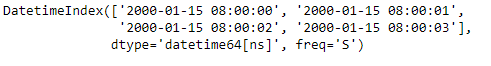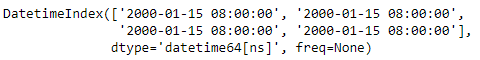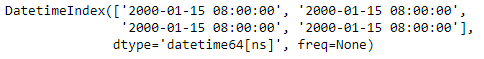# Python | Pandas DatetimeIndex.round()

• Last Updated : 24 Dec, 2018

Python is a great language for doing data analysis, primarily because of the fantastic ecosystem of data-centric python packages. Pandas is one of those packages and makes importing and analyzing data much easier.

Pandas` DatetimeIndex.round()` function localize tz-naive DatetimeIndex to tz-aware DatetimeIndex. This method takes a time zone (tz) naive DatetimeIndex object and makes this time zone aware. It does not move the time to another time zone. Time zone localization helps to switch from time zone aware to time zone unaware objects.

Syntax: DatetimeIndex.round(freq, *args, **kwargs)

Parameters :
freq : The frequency level to round the index to. Must be a fixed frequency like ‘S’ (second) not ‘ME’ (month end)

Return : Index of the same type for a DatetimeIndex or TimedeltaIndex, or a Series with the same index for a Series.

Example #1: Use `DatetimeIndex.round()` function to round the data of the DatetimeIndex object to the specified frequency.

 `# importing pandas as pd``import` `pandas as pd`` ` `# Create the DatetimeIndex``# Here 'S' represents secondly frequency ``didx ``=` `pd.DatetimeIndex(start ``=``'2000-01-15 08:00'``, ``                           ``freq ``=``'S'``, periods ``=` `4``)`` ` `# Print the DatetimeIndex``print``(didx)`

Output :Now we want to convert the second based frequency of the DatetimeIndex object to minute based frequency

 `# convert to the passed frequency``# 'T' represents minute based frequency``didx.``round``(freq ``=``'T'``)`

Output :As we can see in the output, the function has rounded off the values to the desired frequency.

Example #2: Use `DatetimeIndex.round()` function to round the data of the DatetimeIndex object to the specified frequency.

 `# importing pandas as pd``import` `pandas as pd`` ` `# Create the DatetimeIndex``# Here 'T' represents minutely frequency ``didx ``=` `pd.DatetimeIndex(start ``=``'2000-01-15 08:00'``, ``                           ``freq ``=``'T'``, periods ``=` `4``)`` ` `# Print the DatetimeIndex``print``(didx)`

Output :Now we want to convert the minute based frequency of the DatetimeIndex object to hour based frequency

 `# convert to the passed frequency``# Convert minute based frequency to hour based frequency``didx.``round``(freq ``=``'H'``)`

Output :As we can see in the output, the function has rounded off the values to the desired frequency.

My Personal Notes arrow_drop_up